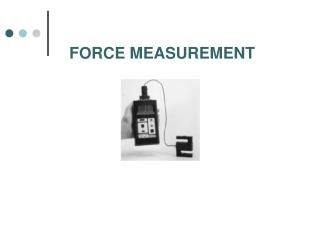DownloadDownload PresentationFORCE MEASUREMENT

# FORCE MEASUREMENT

Download Presentation## FORCE MEASUREMENT

- - - - - - - - - - - - - - - - - - - - - - - - - - - E N D - - - - - - - - - - - - - - - - - - - - - - - - - - -
##### Presentation Transcript

1. FORCE MEASUREMENT

2. INTRODUCTION • TRANSDUCERS THAT MEASURE FORCE AND TORQUE USUALLY • CONTAIN AN ELASTIC MEMBER THAT CONVERTS THE MECHANICAL • QUANTITY TO A DEFLECTION OR STRAIN (IS CALLED LOAD CELL) • A DEFLECTION SENSOR (FOR EXAMPLE, STRAIN GAGE) IS THEN USED • TO GIVE AN ELECTRICAL SIGNAL PROPORTIONAL TO THE QUANTITY • OF INTEREST (FORCE OR TORQUE) • RANGE, LINEARITY AND SENSITIVITY ARE DETERMINED BY THE SIZE • AND SHAPE OF THE ELASTIC MEMBER, THE MATERIAL USED AND • THE SENSOR

3. LOAD CELLS The elastic members commonly used in load cells are links, beam, rings, and shear web

4. LINK TYPE LOAD CELL P Wheatstone bridge strain gage 3(axial) on the back face strain gage 4 (transverse) strain gage 2 (transverse) For full bridge arrangement strain gage 1 (axial) If R1=R2=R3=R4 P

5. EXAMPLE A strip of high strength steel has a length of 30 cm and a cross section of 20 mm by 20 mm. The modulus of elasticity is 200 GPa, and Poisson’s ratio is 0.27. It is subjected to an axial load of 15000 N and it is instrumented with full bridge arrangement of strain gage with R=120 W and gage factor of 2.10. Determine output voltage if a supply voltage of 2.5 V is applied. Solution : Use equation

6. BEAM TYPE LOAD CELL Beam type load cells are commonly employed for measuring low-level loads where the link type load cell is too stiff to be effective P x 1 h 3 b axial gage 1 and 3 If all strain gage are identical axial gage 2 and 4 on the bottom surface

7. TORQUE MEASUREMENT (TORQUE CELLS) Torque cells are transducers that convert an applied torque to an electrical output signal. For circular shaft subjected to pure torsion, all normal stress are 0, the principal stress s1=s2=txz Principal strain

8. MECHANICAL POWER MEASUREMENT (DYNAMOMETER)

9. END OF LECTURE